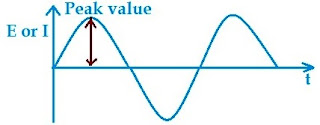Basics

Alternating current

The current whose magnitude and direction both change with time and the same cycle is repeated after a regular interval of time is called alternating current. Thus an alternating quantity (either current or voltage) is one which periodically passes through a definite cycle, each consisting of two half cycles during one of which current or voltage around the circuit varies in one direction and during the other, in opposite direction. Alternators produce an e.m.f. which is for all practice purpose is sinusoidal. As the variation are like sine curve it is called sinusoidal A.C. Such type of a.c. is produced when a coil in a uniform magnetic field with angular velocity ω. The flux associated with coil,
φ = NBA cosωt = NBA cosθ
where θ is the angle covered by normal to plane of coil with B(vector) in time t. Due to change in flux induced emf is,
where E = instantaneous emf, E0 = maximum or peak value of voltage and corresponding current I = I0sinωt.Alternating Quantity waveform

Waveform

Shape of curve of voltage or current when plotted against time as base is called waveform. The waveform of induced e.m.f. in an alternator differs slightly from that of sine wave but for calculation purposes it is treated as such. The advantage of doing so is that calculation becomes simple.

Alternation or Cycle

When a periodic wave such as sinusoidal wave goes through one complete set of positive or negative values, it completes one alternation.
One complete set of positive and negative values of an alternating quantity is known as one cycle. A cycle may also sometimes be specified in terms of angular measure. In that case one complete cycle is said to spread over 360° or 2π radians.

Amplitude

The maximum value, positive or negative, which an alternating quantity attains during one cycle is called the amplitude of the alternating quantity.

Periodic Time or Time Period

The time taken by an alternating quantity to complete one cycle is called the periodic time. Time period is the reciprocal of frequency.
T = 1/ƒ

Frequency

The number of cycles completed per second by an alternating quantity is called frequency.
ƒ = 1/T
In SI system the frequency is expressed in Hertz (Hz). In multipolar machine having P poles and running at a speed of N rpm, frequency of generated emf is,
ƒ = PN/120

Instantaneous Value

Alternating current or voltage changes from instant to instant. The value of alternating current or voltage at any particular instant is called instantaneous value. Instantaneous value of an alternating quantity can be determined either from the curve or from an equation of the alternating quantity.

RMS Value

The rms value of an alternating current is given by that steady (D.C.) current which when flowing through a given circuit for a given time produce the same amount of heat as produced by the alternating current when flown through the same circuit for the same time.
The effective or virtual value of an alternating current or voltage is equal to the square root of the mean of the squares of successive ordinates and that is why it is known as root-mean-square value.
Erms = Emax × 1/√2
The root mean square of effective values for perfect sinusoidal, half wave rectified, full wave rectified, rectangular and triangular wave alternating currents are 0.707, 0.5, 0.707, 1 and 0.578 times the maximum value respectively.

Average or Mean value

The average or mean value of an alternating current is expressed by that steady (D.C.) current which transfers across any circuit the same charge as it is transferred by that of alternating current during the same time. Since in case of a symmetrical alternating current(i.e., one whose two half cycles are exactly similar whether sinusoidal or non sinusoidal) the average mean value over a complete cycle is zero. Hence for such alternating quantities average or mean value is determined by taking the cycle or one alternation only. However for unsymmetrical alternating current the average values means the value determined by taking the mean of instantaneous value over complete cycle.
Eav = 0.636Emax
The average value for perfect sinusoidal, half wave rectified, full wave rectified, rectangular and triangular wave alternating current are 0.636, 0.318, 0.636, 1 and 0.5 times the maximum value respectively. The average value or mean value is only of use in connection with process where the results depend on the current only, irrespective of voltage such as electroplating, or battery charging.

Form factor

It is the ratio of rms value to the average value and denoted by Kf.
Form factor Kf = RMS value/Average value = 0.737Emax/0.636Emax = 1.11
The values of form factor for perfect sinusoidal, half wave rectified, full wave rectified, rectangular and triangular wave alternating current are 1.11, 1.57, 1.11, 1 and 1.6 respectively.
Peak Value
It is the ratio of maximum value to rms value or effective value. It is very essential in connection with determining the dielectric strength since the dielectric stress developed in any insulating material is proportional to the maximum value of voltage applied to it. It is denoted by Kp.
Peak Value = maximum value/rms value
The values of peak or crest factor for perfect sinusoidal, half wave rectified, full wave rectified, rectangular and triangular wave alternating currents are √2, 2, √2, 1 and √3 respectively.